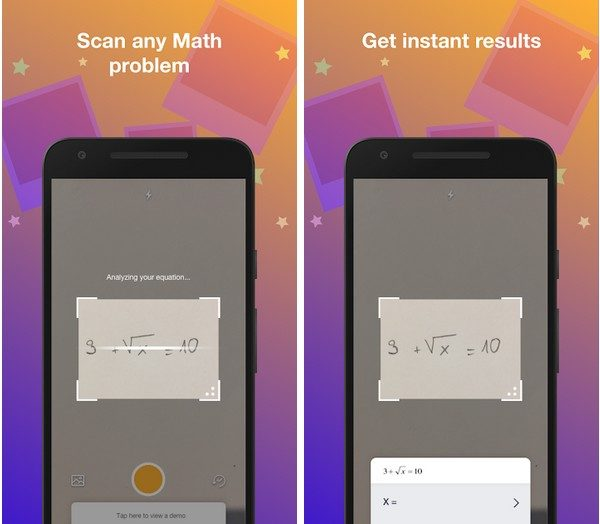Home Education PhotoSolver Instant Math Solutions APK

# PhotoSolver Instant Math Solutions APK

4348
0
SHARENeed help solving a math problem or checking if you have done it correctly? Use Photo Solver to snap a pic and instantly get the solution! Not only that, Photo Solver is 100% FREE!

Get everything you need with Photo Solver:
• No wait, instant math solutions that are 100% FREE
• Step-by-step solutions for different methods to solve the math problem
• Handwriting recognition
• Fast, efficient, and accurate

Photo Solver covers these math subjects:
• Basic Math
• Pre-Algebra
• Algebra
• Linear Algebra
• Trigonometry
• Precalculus
• Calculus
• Finite Math

Requirements: Android 5.0+
Developer: GotIt!